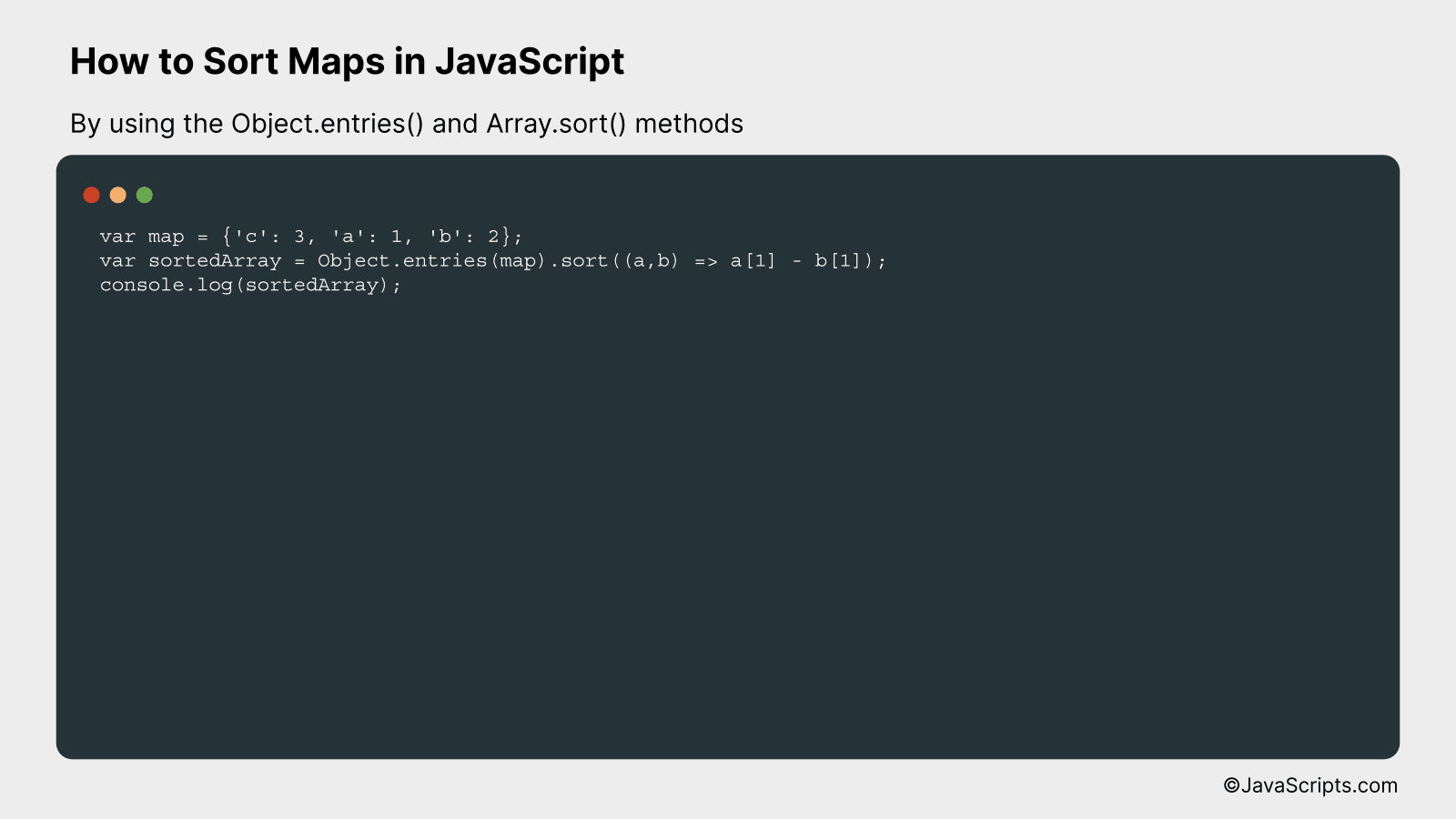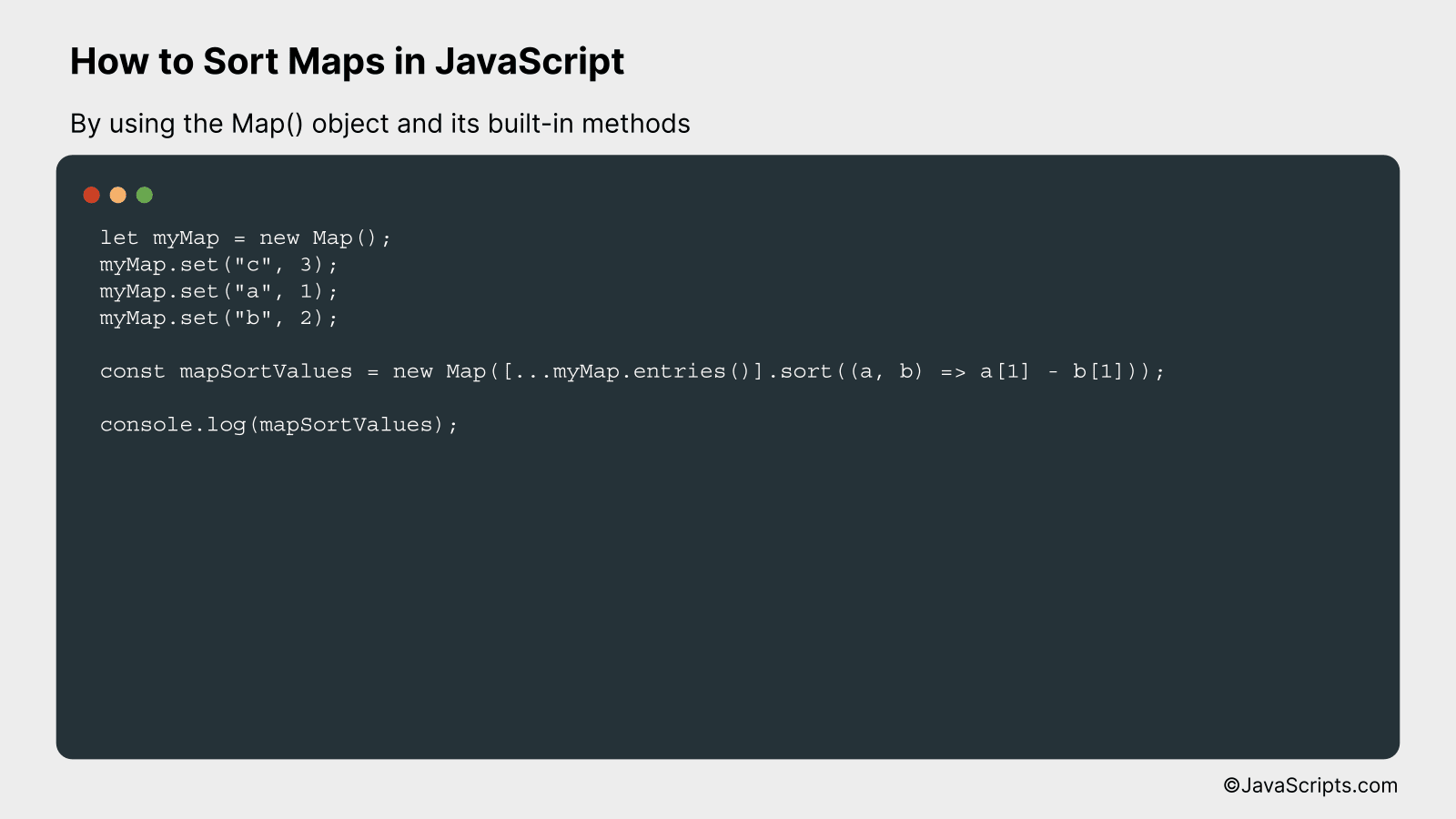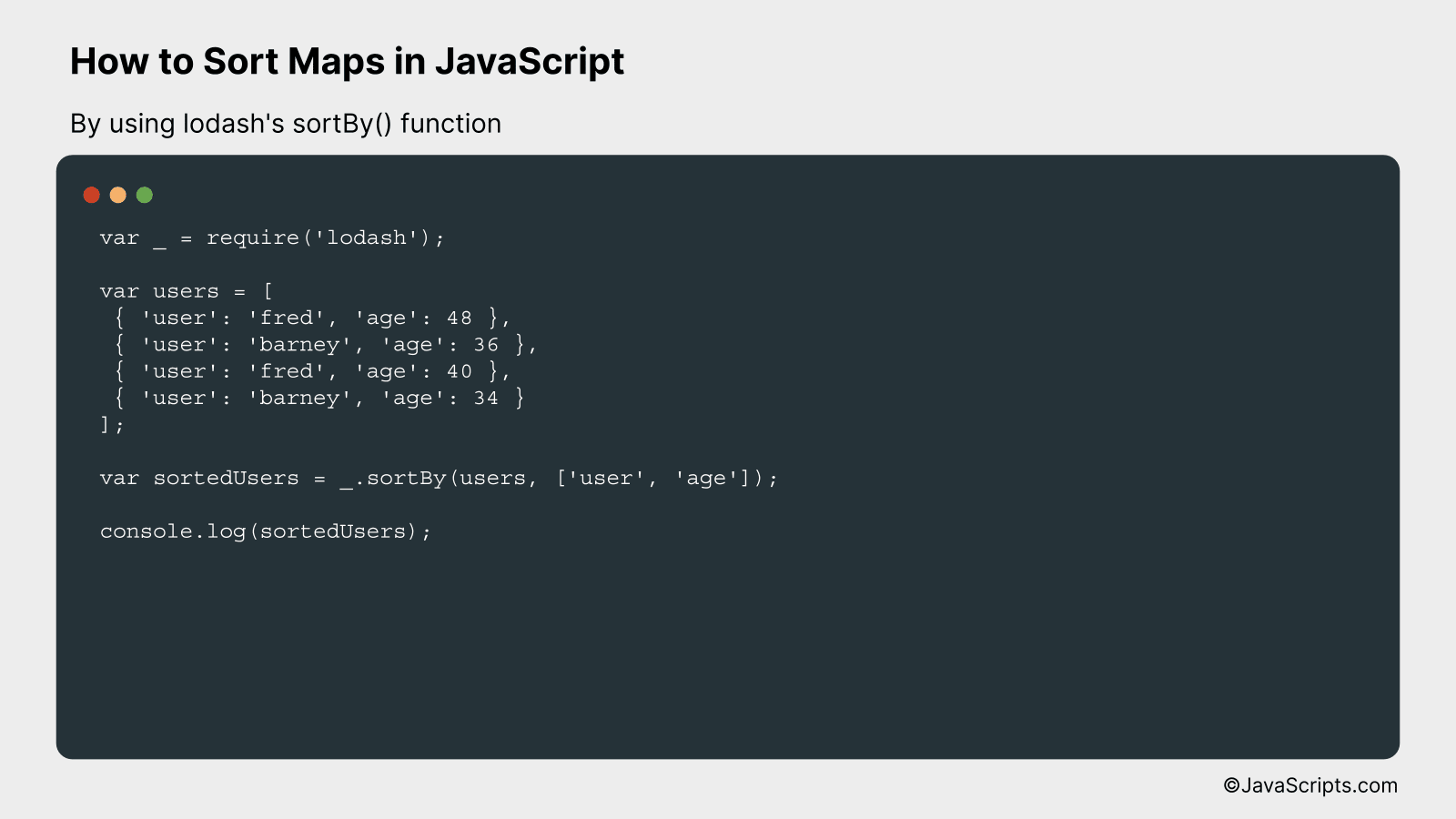# How to Sort Maps in JavaScript

JavaScript is a powerful language, and one of its many features is the ability to handle maps. Maps are key-value pairs which offer a dynamic way to store, access, and organize data. However, when it comes to sorting these maps, things can get a little tricky.

Let’s delve into the nuances of sorting maps in JavaScript. Whether you are a novice or a seasoned pro, understanding this concept can greatly improve your coding skills. Remember, it’s not about complexity; it’s all about simplicity and clarity.

In this post, we will be looking at the following 3 ways to sort maps in JavaScript:

• By using the Object.entries() and Array.sort() methods
• By using the Map() object and its built-in methods
• By using lodash’s sortBy() function

Let’s explore each…

## #1 – By using the Object.entries() and Array.sort() methodsThis method works by transforming the map into an array of key-value pairs using the Object.entries() method, then sorting the resulting array using the Array.sort() method. We will understand this better with an example.

``````
var map = {'c': 3, 'a': 1, 'b': 2};
var sortedArray = Object.entries(map).sort((a,b) => a - b);
console.log(sortedArray);
``````

### How it works

Our map object is converted to an array of arrays, where each inner array contains two items: the key and the value from the map. This array is then sorted based on the values (the second element in each inner array).

• Step 1: First, we define a map object with keys and values. In our case, it’s {‘c’: 3, ‘a’: 1, ‘b’: 2}.
• Step 2: Object.entries(map) is used to convert the map into an array of key-value pairs. This returns an array of arrays, where each inner array has two items: the key and its corresponding value from the map. So the array would look like this: [ [‘c’, 3], [‘a’, 1], [‘b’, 2]].
• Step 3: This array is then sorted using the Array.sort() function. Here, we have a callback function (a,b) => a – b. This will sort the array in ascending order based on the values in the map (i.e., the second items in the inner arrays).
• Step 4: The sorted array is then stored in the sortedArray variable. So, sortedArray will be: [ [‘a’, 1], [‘b’, 2], [‘c’, 3]].
• Step 5: Finally, we print the sortedArray using console.log(sortedArray), which will output the sorted array in the console.

## #2 – By using the Map() object and its built-in methodsWe will be using JavaScript’s built-in Map() object and its methods to sort map entries based on their values, and then we’ll demonstrate this with a simple example where a map is sorted in ascending order based on its values.

``````
let myMap = new Map();
myMap.set("c", 3);
myMap.set("a", 1);
myMap.set("b", 2);

const mapSortValues = new Map([...myMap.entries()].sort((a, b) => a - b));

console.log(mapSortValues);
``````

### How it works

In this code, we use JavaScript’s Map() object to sort the entries based on their values.

1. First, we create a Map object ‘myMap’ and set keys and corresponding values using the ‘set’ method.
2. Then, we create a new Map object ‘mapSortValues’. Here, we use the spread operator ‘…’ to spread out the entries in our ‘myMap’ object. This is done inside an array [] because the Map object’s ‘entries’ method returns a new iterator object that contains an array of [key, value] for each element in the original Map object.
3. We then use the ‘sort’ method to sort these entries. The ‘sort’ method takes a sorting function as an argument, which gets ‘a’ and ‘b’ as parameters. These represent two elements of the array being sorted. In our case, ‘a’ and ‘b’ are also arrays with two elements each – the key and the value from our original map. By returning ‘a – b’, we ensure that the entries are sorted in ascending order of their values.
4. Finally, we log ‘mapSortValues’ to the console to verify our results.

## #3 – By using lodash’s sortBy() functionThe lodash’s sortBy() function is used in JavaScript to sort an array of objects/maps by one or more properties. It receives the array and the property name(s) as arguments, and returns a new array that is sorted by the specified properties. Let’s see it in action with a simple example.

``````
var _ = require('lodash');

var users = [
{ 'user': 'fred', 'age': 48 },
{ 'user': 'barney', 'age': 36 },
{ 'user': 'fred', 'age': 40 },
{ 'user': 'barney', 'age': 34 }
];

var sortedUsers = _.sortBy(users, ['user', 'age']);

console.log(sortedUsers);
```
```

### How it works

The lodash’s sortBy() function sorts the array of objects/maps in ascending order by the properties specified as arguments. In the provided example, the array of users is sorted first by the ‘user’ property, and then by the ‘age’ property.

• First, lodash’s sortBy() function is invoked with the users array and an array of property names [‘user’, ‘age’] as arguments.
• The function sorts the users array first by the ‘user’ property. So, all the objects with ‘user’ as ‘barney’ come before the objects with ‘user’ as ‘fred’.
• Next, within each group of users with the same name, the function sorts the objects by the ‘age’ property. Therefore, the object with ‘user’ as ‘barney’ and ‘age’ as 34 comes before the object with ‘user’ as ‘barney’ and ‘age’ as 36, and so on.
• Finally, the sorted array of objects is stored in the sortedUsers variable and logged to the console.

Related:

In conclusion, sorting maps in JavaScript is a straightforward process that involves transforming a Map into an Array, employing array-sorting methods, and then turning it back into a Map if necessary. This functionality allows developers to handle and display data in a manner that best fits their application’s needs.

Keep in mind that map sorting in JavaScript may vary based on the browser compatibility and the data types you’re working with. Therefore, always test your code thoroughly to ensure it behaves as expected. Remember, practice makes perfect when dealing with complex structures like Map in JavaScript.